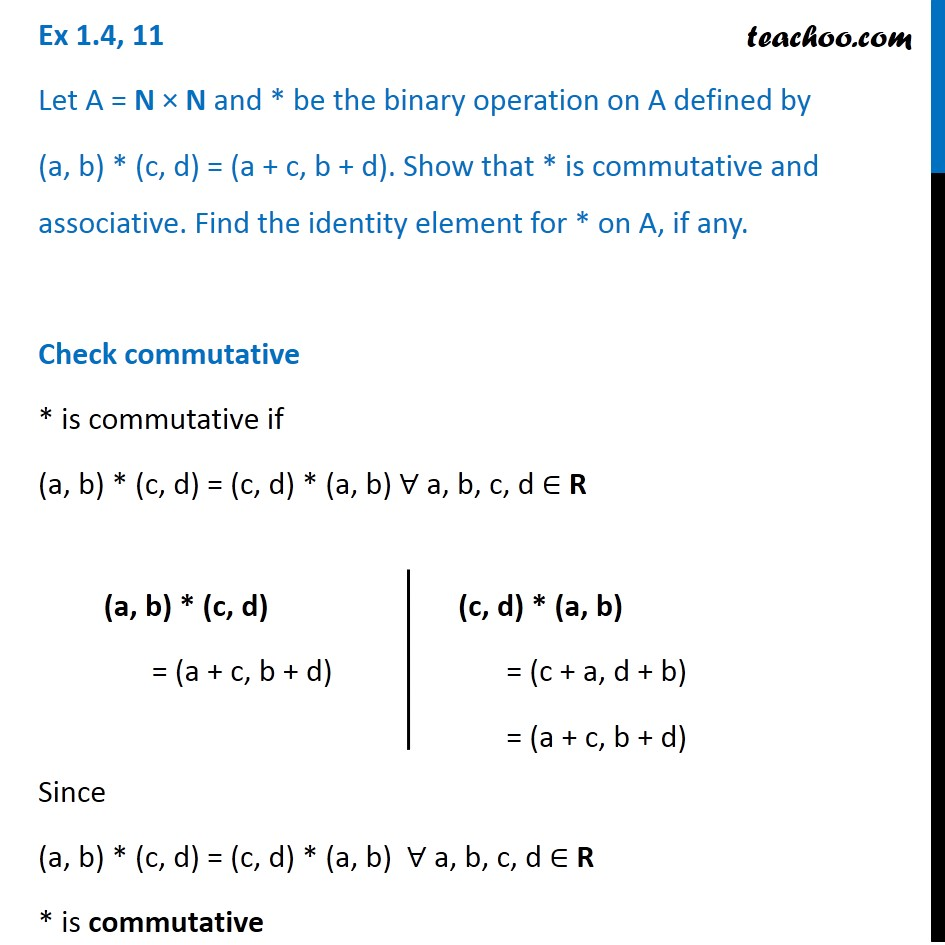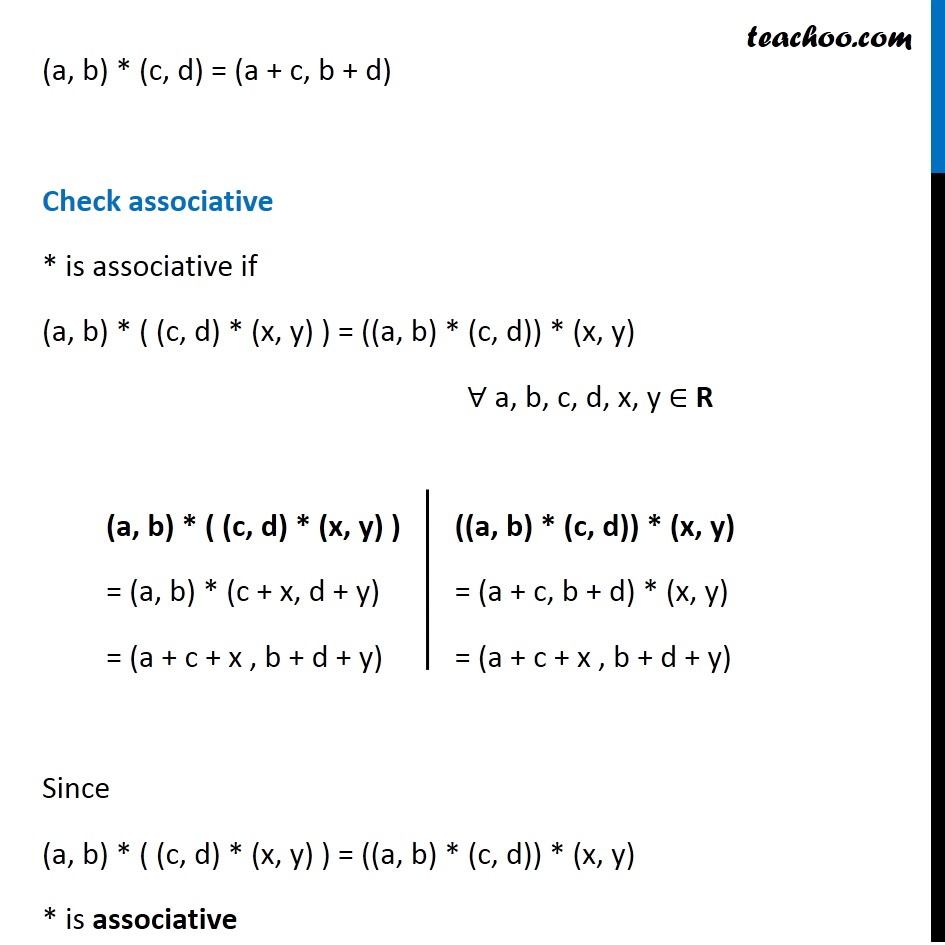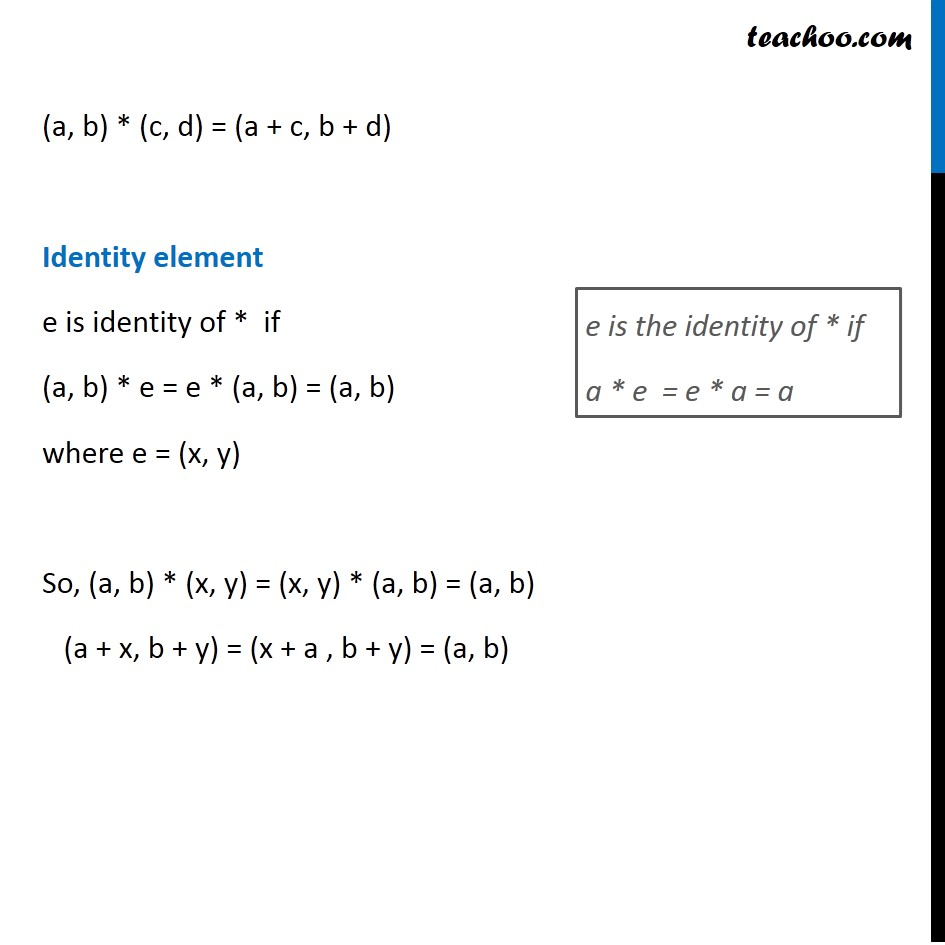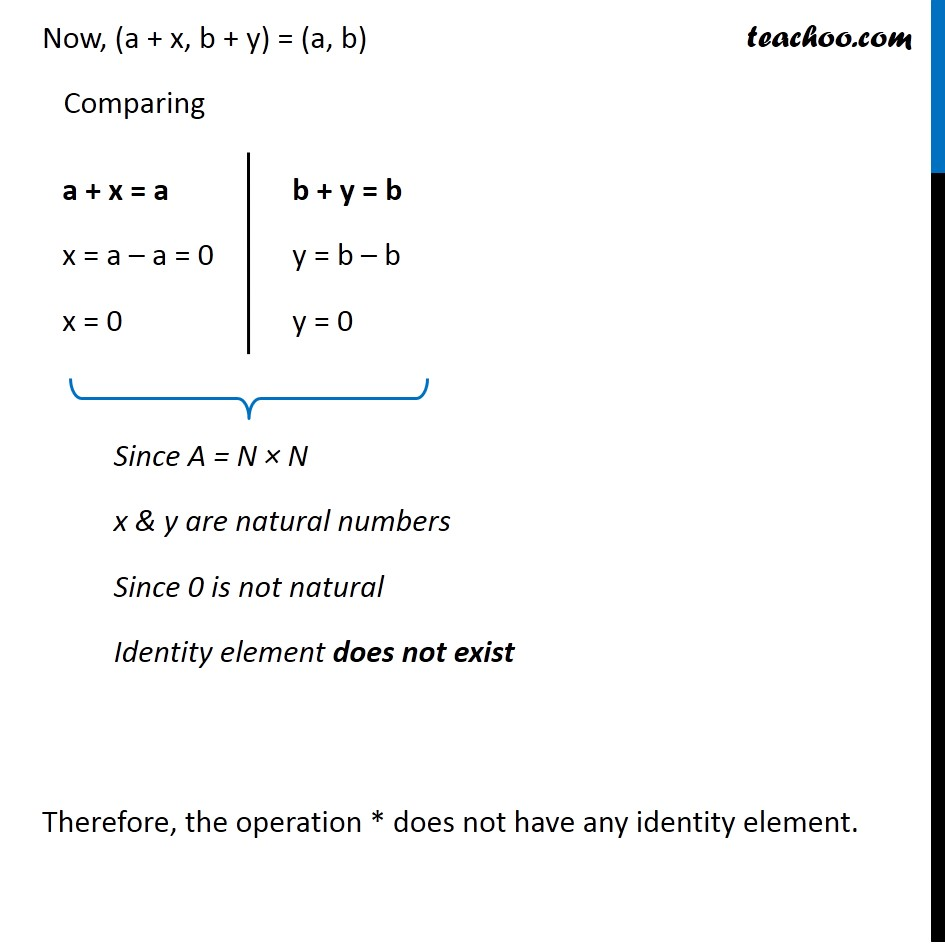Binary Operations

Chapter 1 Class 12 Relation and Functions
Serial order wiseLearn in your speed, with individual attention - Teachoo Maths 1-on-1 Class

### Transcript

Ex 1.4, 11 Let A = N × N and * be the binary operation on A defined by (a, b) * (c, d) = (a + c, b + d). Show that * is commutative and associative. Find the identity element for * on A, if any. Check commutative * is commutative if (a, b) * (c, d) = (c, d) * (a, b) ∀ a, b, c, d ∈ R Since (a, b) * (c, d) = (c, d) * (a, b) ∀ a, b, c, d ∈ R * is commutative (a, b) * (c, d) = (a + c, b + d) (c, d) * (a, b) = (c + a, d + b) = (a + c, b + d) (a, b) * (c, d) = (a + c, b + d) Check associative * is associative if (a, b) * ( (c, d) * (x, y) ) = ((a, b) * (c, d)) * (x, y) ∀ a, b, c, d, x, y ∈ R Since (a, b) * ( (c, d) * (x, y) ) = ((a, b) * (c, d)) * (x, y) * is associative (a, b) * ( (c, d) * (x, y) ) = (a, b) * (c + x, d + y) = (a + c + x , b + d + y) ((a, b) * (c, d)) * (x, y) = (a + c, b + d) * (x, y) = (a + c + x , b + d + y) (a, b) * (c, d) = (a + c, b + d) Identity element e is identity of * if (a, b) * e = e * (a, b) = (a, b) where e = (x, y) So, (a, b) * (x, y) = (x, y) * (a, b) = (a, b) (a + x, b + y) = (x + a , b + y) = (a, b) e is the identity of * if a * e = e * a = a Now, (a + x, b + y) = (a, b) Comparing Therefore, the operation * does not have any identity element. a + x = a x = a – a = 0 x = 0 b + y = b y = b – b y = 0 Since A = N × N x & y are natural numbers Since 0 is not natural Identity element does not exist